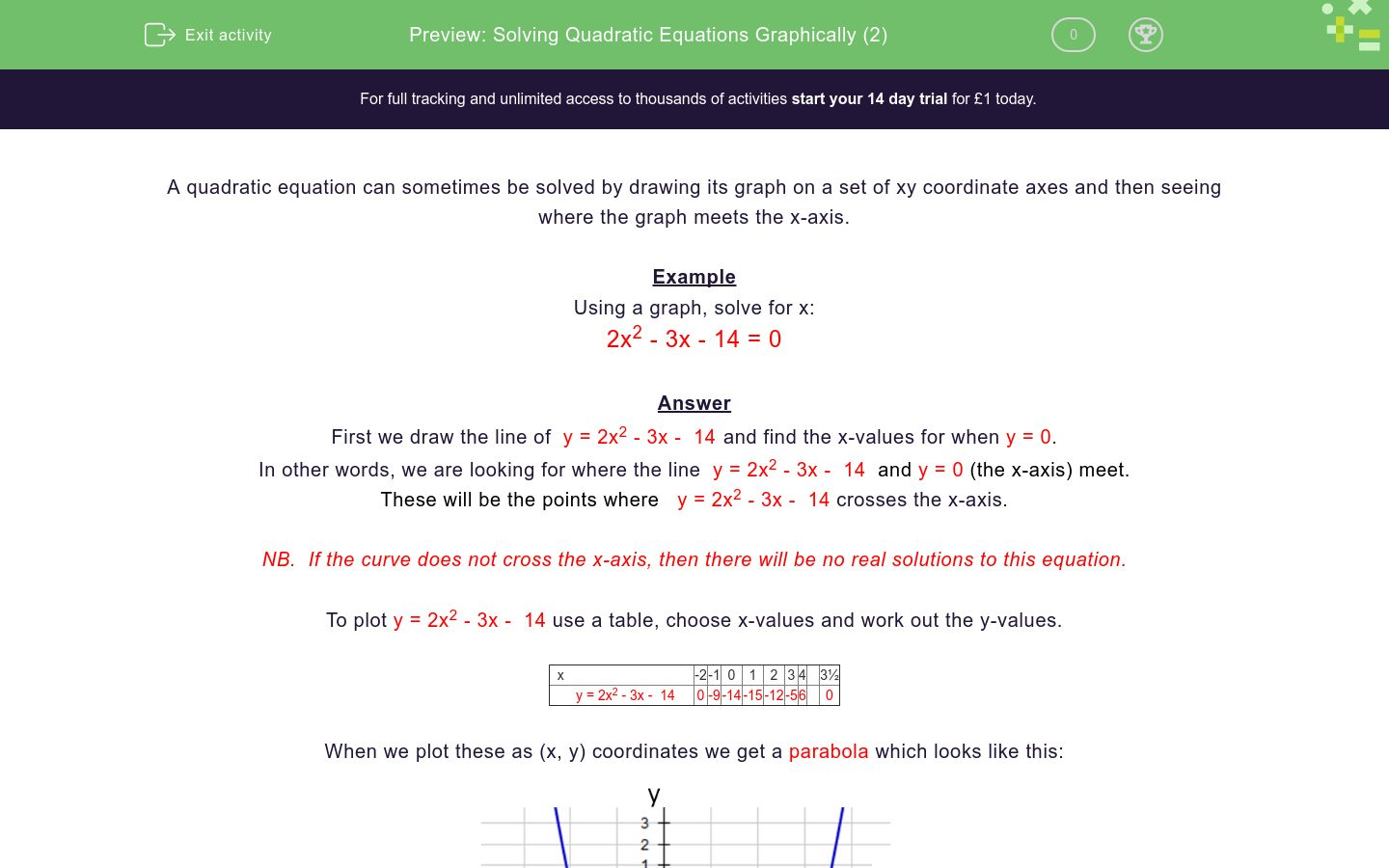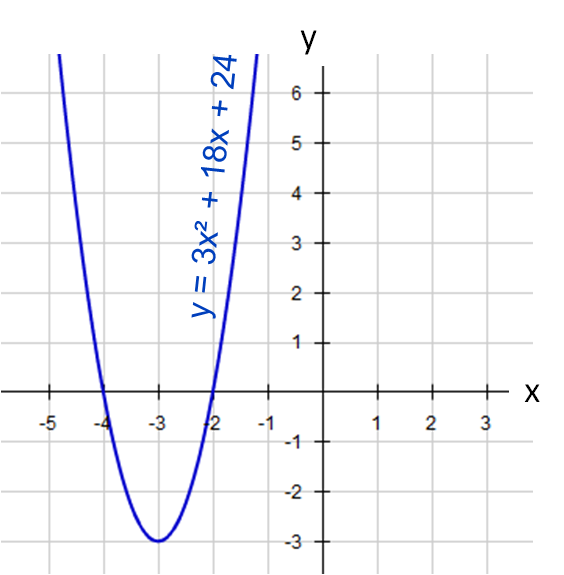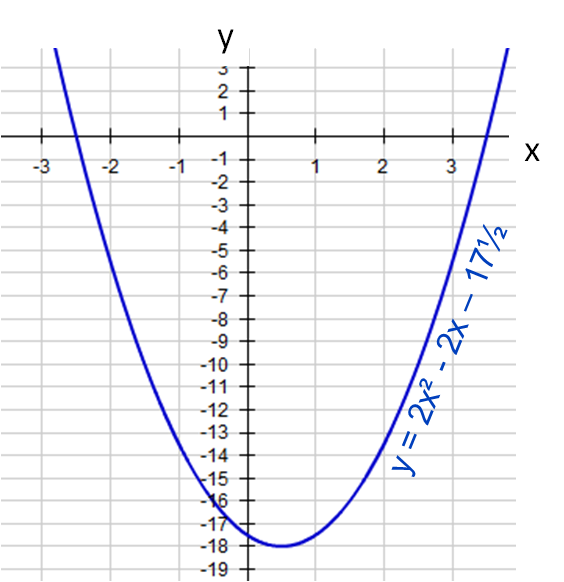# Solving Quadratic Equations Graphically (2)

In this worksheet, students solve quadratic equations by drawing graphs and finding the intercepts on the x-axis.Key stage:  KS 4

Curriculum topic:  Algebra

Curriculum subtopic:  Solve Quadratic Equations by Factorising

Difficulty level:### QUESTION 1 of 10

A quadratic equation can sometimes be solved by drawing its graph on a set of xy coordinate axes and then seeing where the graph meets the x-axis.

Example

Using a graph, solve for x:

2x2 - 3x - 14 = 0

First we draw the line of  y = 2x2 - 3x -  14 and find the x-values for when y = 0.

In other words, we are looking for where the line  y = 2x2 - 3x -  14  and y = 0 (the x-axis) meet.

These will be the points where   y = 2x2 - 3x -  14 crosses the x-axis.

NB.  If the curve does not cross the x-axis, then there will be no real solutions to this equation.

To plot y = 2x2 - 3x -  14 use a table, choose x-values and work out the y-values.

 x -2 -1 0 1 2 3 4 3½ y = 2x2 - 3x -  14 0 -9 -14 -15 -12 -5 6 0

When we plot these as (x, y) coordinates we get a parabola which looks like this:The intercepts on the x-axis are at (-2, 0) and (, 0)

Solution is:

x = -2 or x = 3½

Using a graph, solve for x and select all the solutions to:

2x2 - 7x = 0

x = -3½

x = 3½

x = -7

x = 0

Using a graph, solve for x and select all the solutions to:

3x2 + 18x + 24 = 0

x = -3

x = 4

x = -4

x = -2

Using a graph, solve for x and select all the solutions to:

2x2 + x  = 0

x = -½

x = ½

x = 1

x = 0

Using a graph, solve for x and select all the solutions to:

2x2 + 5x - 3 = 0

x = -1

x = -3

x = ½

x = -5

Using a graph, solve for x and select all the solutions to:

4x2 + 4x + 1 = 0

x = 4

x = -½

x = -2

x = 2

Using a graph, solve for x and select all the solutions to:

5x2 - 35x + 50 = 0

x = 2

x = -7

x = -35

x = 5

Using a graph, solve for x and select all the solutions to:

2x2 + 9x - 5 = 0

x = 5

x = ½

x = -2½

x = -5

Using a graph, solve for x and select all the solutions to:

2x2 + 5x - 12 = 0

x = 4

x = 1½

x = -4

x = -2/3

Using a graph, solve for x and select all the solutions to:

2x2 - 2x - 17½ = 0

x = -5

x = -10

x = -2½

x = 3½

Using a graph, solve for x and select all the solutions to:

2x2 - 5x - 12 = 0

x = -4

x = -3/2

x = 3/2

x = 4

• Question 1

Using a graph, solve for x and select all the solutions to:

2x2 - 7x = 0

x = 3½
x = 0
EDDIE SAYSThe intercepts on the x-axis are at (0, 0) and (, 0)

• Question 2

Using a graph, solve for x and select all the solutions to:

3x2 + 18x + 24 = 0

x = -4
x = -2
EDDIE SAYSThe intercepts on the x-axis are at (-4, 0) and (-2, 0)

• Question 3

Using a graph, solve for x and select all the solutions to:

2x2 + x  = 0

x = -½
x = 0
EDDIE SAYSThe intercepts on the x-axis are at (, 0) and (0, 0)

• Question 4

Using a graph, solve for x and select all the solutions to:

2x2 + 5x - 3 = 0

x = -3
x = ½
EDDIE SAYSThe intercepts on the x-axis are at (-3, 0) and (½, 0)

• Question 5

Using a graph, solve for x and select all the solutions to:

4x2 + 4x + 1 = 0

x = -½
EDDIE SAYSThe graph touches the x-axis at (, 0)

• Question 6

Using a graph, solve for x and select all the solutions to:

5x2 - 35x + 50 = 0

x = 2
x = 5
EDDIE SAYSThe intercepts on the x-axis are at (2, 0) and (5, 0)

• Question 7

Using a graph, solve for x and select all the solutions to:

2x2 + 9x - 5 = 0

x = ½
x = -5
EDDIE SAYSThe intercepts on the x-axis are at (-5, 0) and (½, 0)

• Question 8

Using a graph, solve for x and select all the solutions to:

2x2 + 5x - 12 = 0

x = 1½
x = -4
EDDIE SAYSThe intercepts on the x-axis are at (-4, 0) and (, 0)

• Question 9

Using a graph, solve for x and select all the solutions to:

2x2 - 2x - 17½ = 0

x = -2½
x = 3½
EDDIE SAYSThe intercepts on the x-axis are at (-2½, 0) and (, 0)

• Question 10

Using a graph, solve for x and select all the solutions to:

2x2 - 5x - 12 = 0

x = -3/2
x = 4
EDDIE SAYSThe intercepts on the x-axis are at (-1½, 0) and (4, 0)

---- OR ----

Sign up for a £1 trial so you can track and measure your child's progress on this activity.

### What is EdPlace?

We're your National Curriculum aligned online education content provider helping each child succeed in English, maths and science from year 1 to GCSE. With an EdPlace account you’ll be able to track and measure progress, helping each child achieve their best. We build confidence and attainment by personalising each child’s learning at a level that suits them.